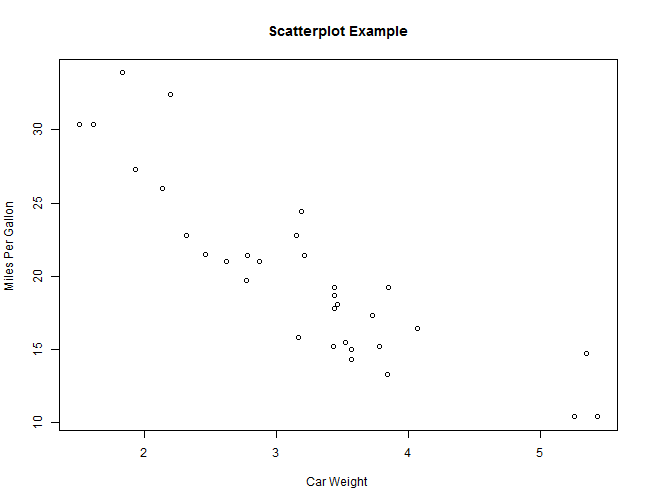# Basic Charts in R

### February 26, 2014 | Alan

This post demonstrates basic charts in R; specifically simple, stacked and grouped bar charts, line charts and scatter plots. Using the built-in R dataset `mtcars`, each chart is derived from a simple data structure, the format of which is also explained.

### Learning Outcomes

The following functions are used in this example:

• `barplot()` plotting function used for bars
• `plot()` plotting function used for lines and scatter.

### Deriving Data for a Simple Bar Chart

The following code derives the counts of cars having a particular number of gears from `mtcars`:

``````data(mtcars)
counts1 <- table(mtcars\$gear)
counts1
``````
``````##
##  3  4  5
## 15 12  5
``````

The resulting dataset is named `counts1` and looks like this: the top row shows the number of gears, the bottom row shows the number of cars with each number of gears.

### Using R Code to Create a Simple Bar Chart

Here we are going to plot bars representing the number of gears on x-axis with the counts of cars having a particular number of gears on the y-axis.
The following code plots this data as a bar chart. Note that:

• `counts1` refers to the dataset
• `main` used to format a plot title
• `xlab` formats the the x-axis label
• `ylab` formats the y-axis label.
``````barplot(counts1, main = "Car Distribution", xlab = "Number of Gears", ylab = "Count")
``````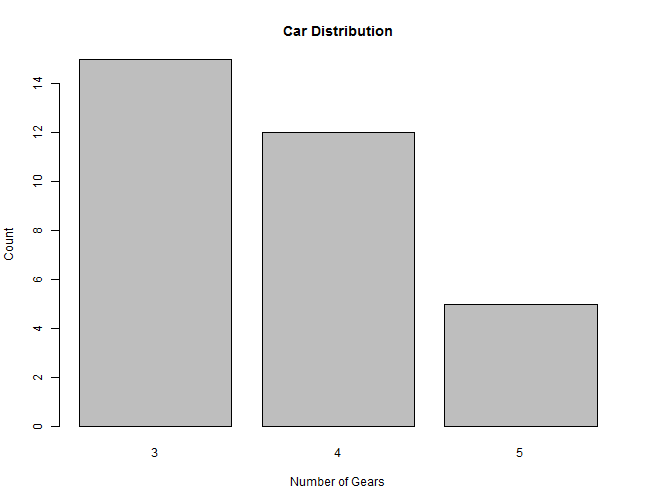### Deriving Data for Stacked Bar Chart

The following code derives data from `mtcars` to show the counts of cars having a particular number of gears, grouped to distinguish cars configured with a V-shaped engine (`vs = 0`) compared to cars configured with a straight engine (`vs = 1`).
The resulting dataset `counts2` looks like this:

``````counts2 <- table(mtcars\$vs, mtcars\$gear)
counts2
``````
``````##
##      3  4  5
##   0 12  2  4
##   1  3 10  1
``````

The top row shows the number of `gears`, the second row shows the counts of each of the gear values with `vs = 0`, and the bottom row shows values with `vs = 1`.

### Creating a Stacked Bar Chart

The following code plots this data as a stacked bar chart. In this case we add a `legend()` for `vs`:

``````barplot(counts2, main = "Car Distribution by Gears and Engine Configuration",
xlab = "Number of Gears", ylab = "Count", legend = rownames(counts2))
``````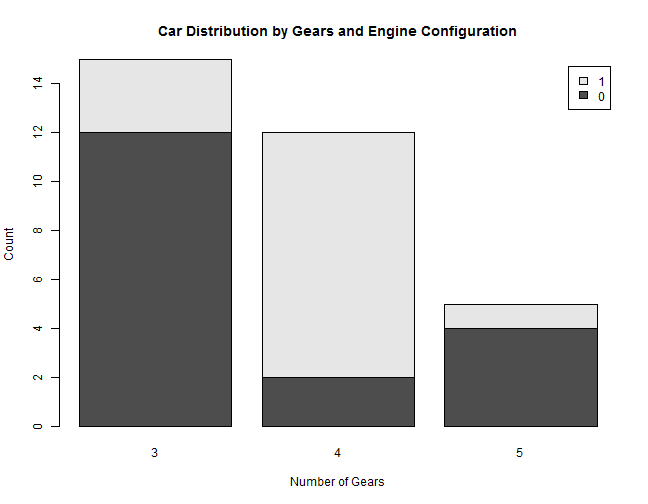### Creating a Grouped Bar Chart

Using the same data as Stacked Bar Chart, the following code plots this data as a Grouped Bar Chart. The change from stacked to grouped is made by setting the argument `beside=TRUE`:

``````barplot(counts2, main = "Car Distribution by Gears and Engine Configuration",
xlab = "Number of Gears", ylab = "Count", legend = rownames(counts2), beside = TRUE)
``````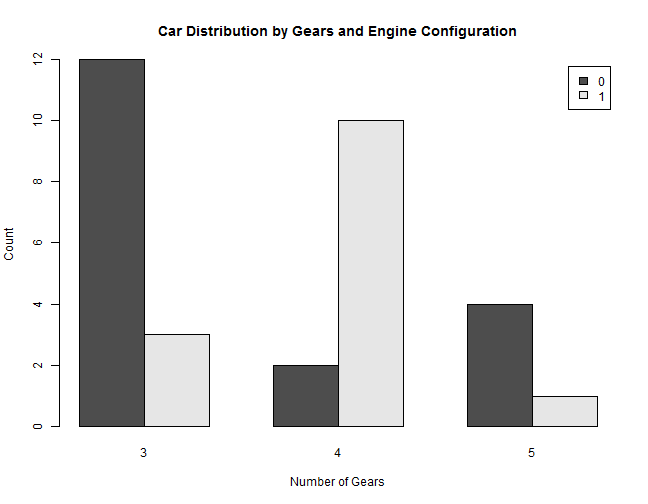### Deriving Data for a Simple Line Chart

For each row of data in the table, this chart plots the weight (`wt`) values sequentially.

Here the data is the `mtcars\$wt` value from `mtcars`. A sample of the data and the code to produce it is as follows:

``````head(mtcars["wt"])
``````
``````##                      wt
## Mazda RX4         2.620
## Mazda RX4 Wag     2.875
## Datsun 710        2.320
## Hornet 4 Drive    3.215
## Hornet Sportabout 3.440
## Valiant           3.460
``````

### Creating a Simple Line Chart

Using the `plot()` function, the following code will plot this data as a line; we denote this line as a line by setting `type = "l"`:

``````plot(mtcars\$wt, type = "l", ylab = "Weight", xlab = "Row Number", main = " Line Example")
``````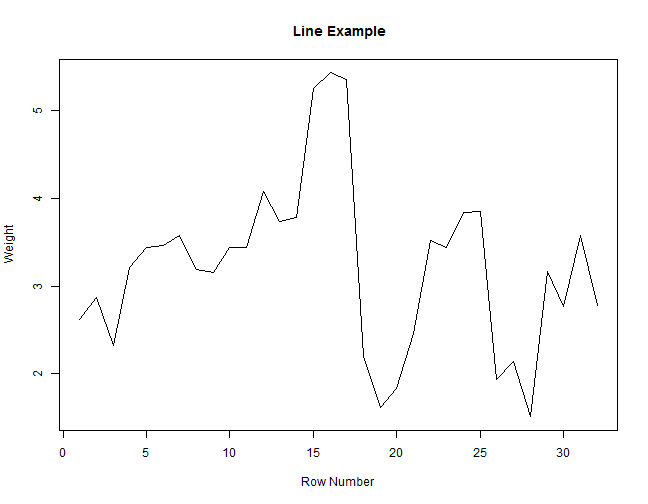### Deriving Data for the Scatter Plot

Using the `plot()` function from the previous example, we introduce a `y` variable `mtcars\$mpg` to produce the values for the scatter plot. A sample of the data and the code to produce it is as follows:

``````head(mtcars[c("wt", "mpg")])
``````
``````##                      wt  mpg
## Mazda RX4         2.620 21.0
## Mazda RX4 Wag     2.875 21.0
## Datsun 710        2.320 22.8
## Hornet 4 Drive    3.215 21.4
## Hornet Sportabout 3.440 18.7
## Valiant           3.460 18.1
``````

### Creating a Scatter Plot

This following code shows the weight (`wt`) of each car compared to the miles per gallon (`mpg`):

``````plot(mtcars\$wt, mtcars\$mpg, main = "Scatterplot Example", xlab = "Car Weight ",
ylab = "Miles Per Gallon ")
``````